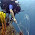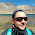## Friday, March 18, 2016

### SiS Repost: Monte Carlo methods, nonparametric tests and you

Often when people first are introduced to ENMTools, a point of confusion arises when they come to the point of having to compare their empirical observations to a null distribution: it’s not something they’ve done so explicitly before, and they’re not quite sure how to do it.  In this post I’m going to try to explain in the simplest possible terms how hypothesis testing, and in particular nonparametric tests based on Monte Carlo methods, work.

Let’s say we’ve got some observation based on real data.  In our case, we’ll say it’s a measurement of niche overlap between ENMs built from real occurrence points for a pair of species (figure partially  adapted (okay, stolen) from a figure by Rich Glor).  We have ENMs for two species, and going grid cell by grid cell, we sum up the differences between those ENMs to calculate a summary statistic measuring overlap, in this case D.
Due to some evolutionary or ecological question we’re trying to answer, we’d like to know whether this overlap is what we’d expect under some null hypothesis.  For the sake of example, we’ll talk about the “niche identity” test of Warren et al. 2008.  In this case, we are asking whether the occurrence points from two species are effectively drawn from the same distribution of environmental variables.  If that is the case, then whatever overlap we see between our real species should be statistically indistinguishable from the overlap we would see under that null hypothesis.  But how do we test that idea quantitatively?

In the case of good old parametric statistics, we would do that by comparing our empirical measurement to a parametric estimate of the overlap expected between two species (i.e., we would say "if the null hypothesis is true, we would expect an overlap of 0.5 with a standard deviation of .05", or something like that).  That would be fine if we could accurately make a parametric estimate of the expected distribution of overlaps under that null hypothesis, i.e., we need to be able to specify a mean and variance for expected overlap under that null hypothesis.  How do we do that?  Well, unfortunately, in our case we can’t.  For one thing we simply can’t state that null in a manner that makes it possible for us to put numbers on those expectations.  For another, standard parametric statistics mostly require the assumption that the distribution of expected measurements under the null hypothesis meets some criteria, the most frequent being that the distribution is normal.  In many cases we don’t know whether or not that’s true, but in the case of ENM overlaps we know it’s probably not true most of the time.  Overlap metrics are bound between 0 and 1, and if the null hypothesis generates expectations that are near one of those extremes, the distribution of expected overlaps is highly unlikely to be even approximately normal.  There can also be (and this is based on experience), multiple peaks in those null distributions, and a whole lot of skew and kurtosis as well.  So a specification of our null based on a normal distribution would be a poor description of our actual expectations under the null hypothesis, and as a result any statistical test based on parametric stats would be untrustworthy.  I have occasionally been asked whether it’s okay to do t-tests or other parametric tests on niche overlap statistics, and, for the reasons I’ve just listed, I feel that the answer has to be a resounding “no”.

So what’s the alternative?  Luckily, it’s actually quite easy.  It’s just a little less familiar to most people than parametric stats are, and requires us to think very precisely about the ideas we’re trying to test.  In our case, what we need to do is to find some way to estimate the distribution of overlaps expected between a pair of species using this landscape and these sample sizes if they were effectively drawn from the same distribution of environments.  What would that imply?  Well, if each of these sets of points were drawn from the same distribution, we should be able to generate overlap values similar to our empirical measurement by repeating that process.  So that’s exactly what we do!

We take all of the points for these two species and we throw them in a big pool.  Then we randomly pull out points for two species from that pool, keeping the sample sizes consistent with our empirical data.  Then we build ENMs for those sets of points and measure overlaps between them.  That gives us a single estimate of expected overlaps under the null hypothesis.  So now we’ve got our empirical estimate (red) and one realization of the null hypothesis (blue)
All right, so it looks like based on that one draw from the null distribution, our empirical overlap is a lot lower than you’d expect.  But how much confidence can we have in this conclusion can we have based on one single draw from the null distribution?  Not very much.  Let’s do it a bunch more times and make a histogram:
All right, now we see that, in 100 draws from that null distribution, we never once drew an overlap value that was as low as the actual value that we get from our empirical data.  This is pretty strong evidence that, whatever process generated our empirical data, it doesn’t look much like the process that generated that null distribution, and based on this evidence we can statistically reject that null hypothesis.  But how do we put a number on that?  Easy!  All we need to do is figure out what the percentile in that distribution is that corresponds to our empirical measurement.  In this case our empirical value is lower than the lowest number in our null distribution.  That being the case, we can’t specify exactly what the probability of getting our empirical result is, only that it’s lower than the lowest value we obtained, so it’s p < (whatever that number is).  Since we did 100 iterations of that null hypothesis (and since our empirical result is also a data point), the resolution of our null distribution is 1/(100 + 1) ~= .01.  Given our resolution, that means p is between 0 and .01 or, as we normally phrase it, p < .01.  If we’d done 500 simulation runs and our empirical value was still lower than our lowest value, it would be p < 1/(500 + 1), or p < .0002.  If we’d done 500 runs and found that our empirical value was between the lowest value and the second lowest value, we would know that .0002 < p < .0004, although typically we just report these things as p < .0004.  Basically the placement of our empirical value in the distribution of expected values from our null hypothesis is an estimate of the probability of getting that value if that hypothesis were true.  This is exactly how hypothesis testing works in parametric statistics, the only difference being that in our case we generated the null distribution from simulations rather than specifying it mathematically.

So there you go!  We now have a nonparametric test of our hypothesis.  All we had to do was (1) figure out precisely what our null hypothesis was, (2) devise a way to generate the expected statistics if that hypothesis were true, (3) generate a bunch of replicate realizations of that null hypothesis to get an expected distribution under that null, and (4) compare our empirical observations to that distribution.  Although this approach is certainly less easy than simply plugging your data into Excel and doing a t-test or whatnot, there are many strengths to the Monte Carlo approach. For instance, we can use this approach to test pretty much any hypothesis that we can simulate – as long as we can produce summary statistics from a simulation that are comparable to our empirical data, we can test the probability of observing our empirical data under the set of assumptions that went into that simulated data.  It also means we don’t have to make assumptions about the distributions that we’re trying to test – by generating those distributions directly and comparing our empirical results to those distributions, we manage to step around many of the assumptions that can be problematic for parametric statistics.

The chief difficulty in applying this method is in steps 2 and 3 above – we have to be able to explicitly state our null hypothesis, and we have to be able to generate the distribution of expected measurements under that null.  Honestly, though, I think this is actually one of the greatest strengths of Monte Carlo methods: while this process may be more intensive than sticking our data into some plug-and-chug stats package, it requires us to think very carefully about what precisely our null hypothesis means, and what it means to reject it.  It requires more work, but more importantly it requires a more thorough understanding of our own data and hypotheses.

1.Hi,
I have been looking to download the latest version of ENMTools. however the folder in above link is without the .exe file. may I know where can I get the .exe file?
thanking you

2.There hasn't been an .exe included with ENMTools for several years now. It's a Perl script, you'll need to download and install Activestate Perl to use it.

3.Thanks for the explanation, is illustrative.